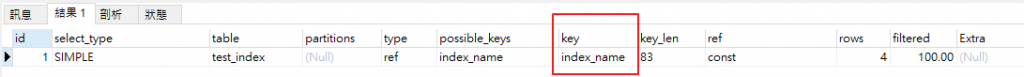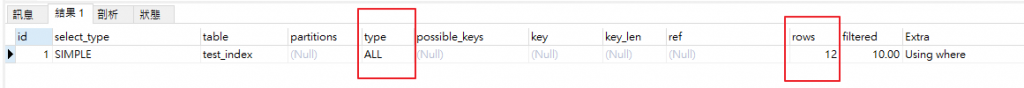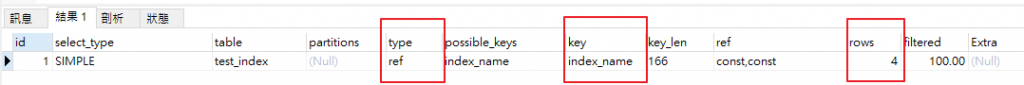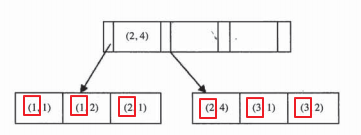1

# 前言

## 複合索引

★ 前提: 不同版本優化器是有差異的，這個版本會幫你優化語法，另一個版本可能不能幫你優化語法。所以這裡會提到一些觀念，可能在新一點的 MySQL 版本會發現不是這麼回事，所以有時間還是要自己實際測試看看。

PK: Id

``````-- 建立測試 TABLE
CREATE TABLE `test_index` (
`Id` int(11) NOT NULL AUTO_INCREMENT,
`A` varchar(20) DEFAULT NULL,
`B` varchar(20) DEFAULT NULL,
`C` varchar(20) DEFAULT NULL,
`D` varchar(20) DEFAULT NULL,
PRIMARY KEY (`Id`),
KEY `index_name` (`A`,`B`,`C`)
) ENGINE=InnoDB

-- 插入資料
INSERT INTO `test_index`(`Id`, `A`, `B`, `C`, `D`) VALUES (1, 'a', 'b', 'c', 'd');
INSERT INTO `test_index`(`Id`, `A`, `B`, `C`, `D`) VALUES (2, 'aa', 'bb', 'cc', 'dd');
INSERT INTO `test_index`(`Id`, `A`, `B`, `C`, `D`) VALUES (3, 'aaa', 'bbb', 'ccc', 'ddd');
INSERT INTO `test_index`(`Id`, `A`, `B`, `C`, `D`) VALUES (4, 'a', 'b', 'c', 'd');
INSERT INTO `test_index`(`Id`, `A`, `B`, `C`, `D`) VALUES (5, 'aa', 'bb', 'cc', 'dd');
INSERT INTO `test_index`(`Id`, `A`, `B`, `C`, `D`) VALUES (6, 'aaa', 'bbb', 'ccc', 'ddd');
INSERT INTO `test_index`(`Id`, `A`, `B`, `C`, `D`) VALUES (7, 'a', 'b', 'c', 'd');
INSERT INTO `test_index`(`Id`, `A`, `B`, `C`, `D`) VALUES (8, 'aa', 'bb', 'cc', 'dd');
INSERT INTO `test_index`(`Id`, `A`, `B`, `C`, `D`) VALUES (9, 'aaa', 'bbb', 'ccc', 'ddd');
INSERT INTO `test_index`(`Id`, `A`, `B`, `C`, `D`) VALUES (10, 'a', 'b', 'c', 'd');
INSERT INTO `test_index`(`Id`, `A`, `B`, `C`, `D`) VALUES (11, 'aa', 'bb', 'cc', 'dd');
INSERT INTO `test_index`(`Id`, `A`, `B`, `C`, `D`) VALUES (12, 'aaa', 'bbb', 'ccc', 'ddd');
``````

##### ◎查詢1
``````EXPLAIN
SELECT *
FROM test_index
WHERE A='a'
``````• type: ref 表示有用到非唯一性索引
• key: index_name 表示用到我們建立的輔助索引
• rows: 4 表示掃描了 4行
##### ◎查詢2
``````EXPLAIN
SELECT *
FROM test_index
WHERE  B='b'
``````• type: ALL 表示沒有用到索引，全表掃描
• rows: 12 整張表的資料都掃了
##### ◎查詢3
``````EXPLAIN
SELECT *
FROM test_index
WHERE  A='a' and B='b'
``````• type: ref 表示有用到非唯一性索引
• key: index_name 表示用到我們建立的輔助索引
• rows: 4 表示掃描了 4行

### 小結

• 事實上當我們輔助索引建立的是 (A,B,C)，會用到索引的情況為
(A), (A,B), (A,C), (A,B,C)
• 不會用到索引的情況為
(B), (C), (B,C)
• 所以如果我們的 WHERE 條件會用到 (A) 欄位也會用到 (A,B) 欄位，表示我們只要建一個 KEY (A,B) 就好，假如此時還有另一個索引 KEY (A)，那麼這個就是多建的，可以刪掉
• 這裡我們還要了解另一件原理，也就是最左前綴原理

## 最左前綴(最左匹配)

MySQL 在建立複合索引的時候會符合最左前綴匹配，也就是說在建立此複合索引的 B+樹時會依照左到右的順序來建

``````CREATE TABLE t (
a INT,
b INT,
PRIMARY KEY (a),
KEY idx_a_b (a,b)
)ENGINE=INNODB
``````• 基於最左前綴，可以發現會先依照欄位 a 來排，所以此 B+樹的順序可以看到由 1 -> 3
• 如果不看 a 只看 b，會發現 b的數字依序是 1,2,1,4,1,2 這樣就沒有順序了
• 所以如果 b 也要有用到索引，那麼 a 欄位也要放進去才可以，也就是當 a 欄位相同時，b欄位就有順序

## 為什麼需要複合索引?

1. 減少硬碟空間占用: 如同前面提到的，假如我們的複合索引(A,B)，搜尋條件有
`where A = xxx`
`where A =xxx and B =xxx`
那麼不用建例兩個索引 (A) 和 (A,B)。不要忘記，一個索引就是一個B+樹
2. 可以使用覆蓋索引: 假設有一個語法 `SELECT A,B,C FROM table_name WHERE A = xxx and B = xxx` ，此時我們有一個複合索引(A,B,C)，表示可以不用回到叢集索引去就可以取得資料。忘記什麼是覆蓋索引可以回到我的上一篇參考 MySQL 系列文 - 索引的相關知識 - 覆蓋索引
3. 第二個欄位已排序: 有時候我們用了 A 欄位來當 WHERE 條件，並且 order by B ，例如 `SELECT * FROM table_name WHERE A = xxx ORDER BY B` 此時選擇了複合索引，B 欄位本身就是排序好的，不用另外排序了。

# 小結

### 1 則留言

0

Stock iT邦新手 5 級 ‧ 2020-03-11 12:29:21 檢舉